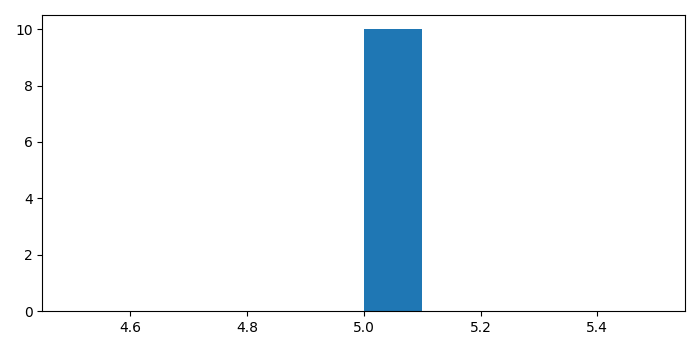# How to normalize a histogram in Python?

PythonMatplotlibServer Side ProgrammingProgramming

#### Beyond Basic Programming - Intermediate Python

Most Popular

36 Lectures 3 hours

#### Practical Machine Learning using Python

Best Seller

91 Lectures 23.5 hours

#### Practical Data Science using Python

22 Lectures 6 hours

To normalize a histogram in Python, we can use hist() method. In normalized bar, the area underneath the plot should be 1.

## Steps

• Make a list of numbers.

• Plot a histogram with density=True.

• To display the figure, use show() method.

## Example

import matplotlib.pyplot as plt

plt.rcParams["figure.figsize"] = [7.00, 3.50]
plt.rcParams["figure.autolayout"] = True

k = [5, 5, 5, 5]
x, bins, p = plt.hist(k, density=True)

plt.show()

## Output